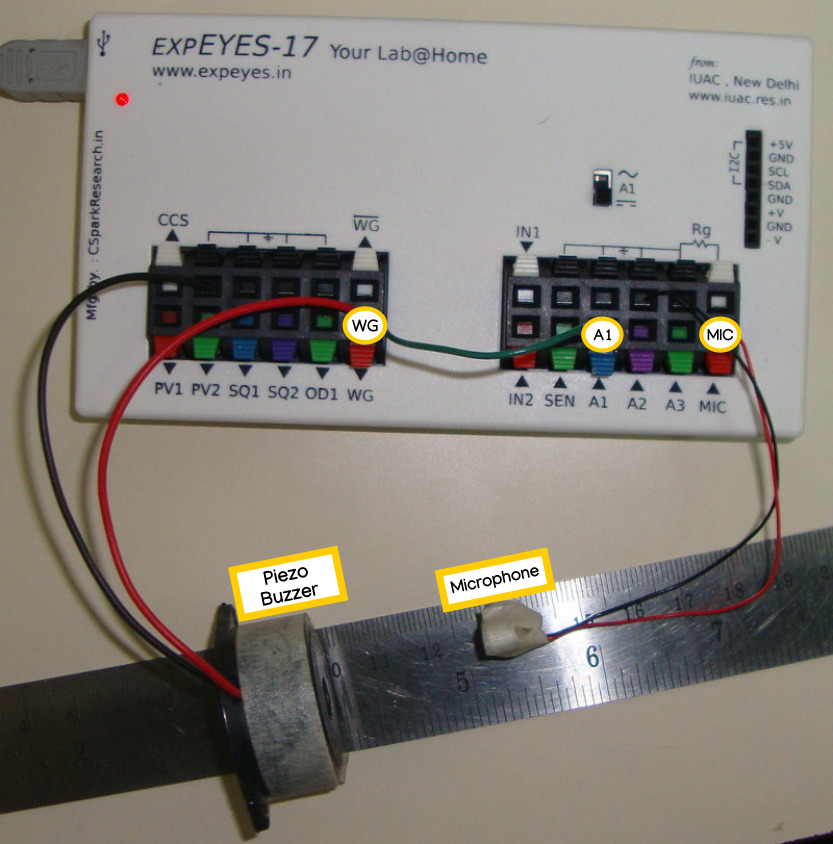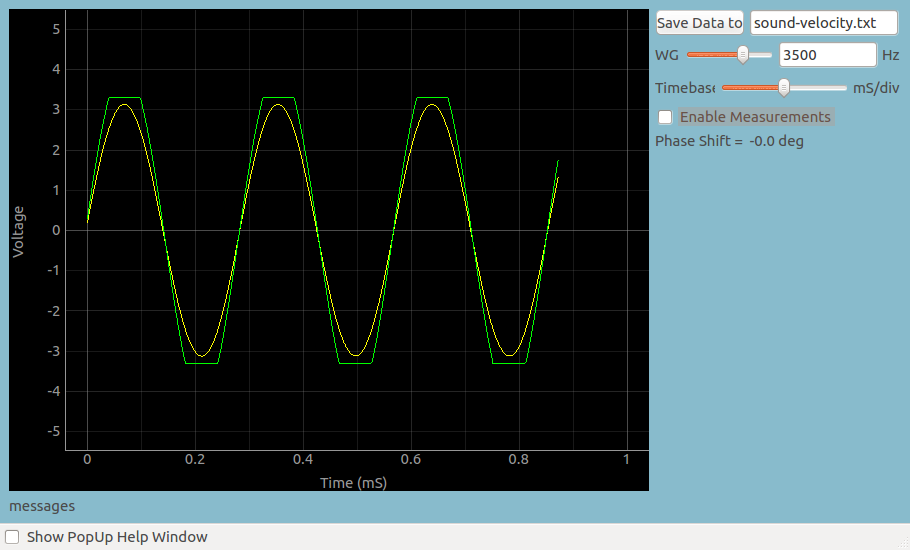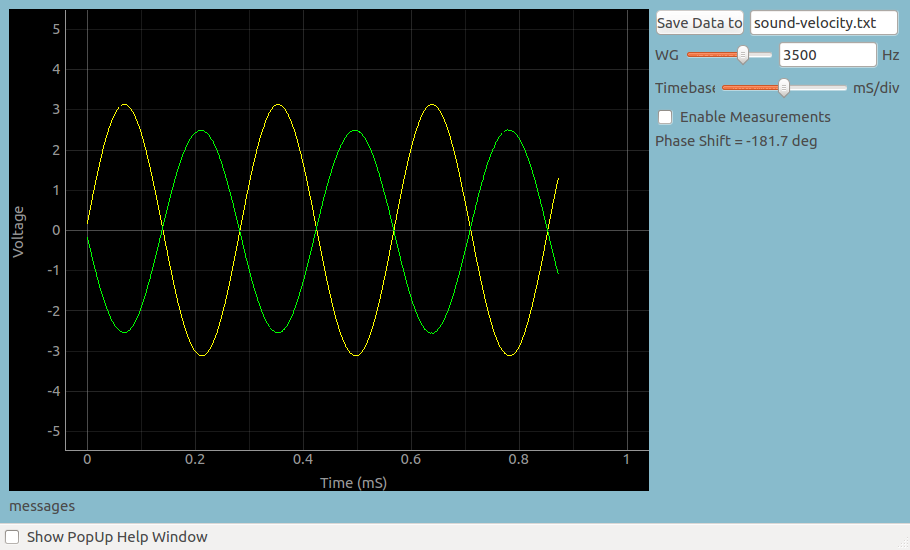# Velocity of Sound

## Measuring the velocity of sound using Phase difference at difference points of propagationBrief Summary
This experiment is aimed at understanding the physics behind sound, namely, the movement of sound through air as a series of compressions and rarefactions.

Sound is digitized by a microphone, and the signal is compared with the oscillating voltage source fed to a buzzer which created the sound. A study of phase shifts using mathematical analysis is used to calculate the velocity of sound in air.

## Introduction

The frequency waveform generator WG is set to the resonant frequency of the Piezo, for maximum sound output. This is around 3500Hz, and is very shrill.

The compressions and expansions of the Piezo generate high and low pressure regions in front of it, which travel at the speed of sound, which in air at 25 degree celcius is around 340m/S .

The microphone digitizes the pressure and plots it as a function of time. Since the applied waveform is sinusoidal, the detected signal will also be sinusoidal in shape. However, Since the compressions and rarefactions of air take some time to reach the microphone, there will be a phase difference between the applied signal and the received signal.The oscilloscope monitors two signals:

• the sine wave driving the Piezo. This is done by connecting WG output to A1.
• and the signal detected by the microphone.

It is triggered by the sine wave driving the Piezo

When the microphone is moved, the phase difference between the two waveforms changes. For half wavelength the phase difference is 180 degree. A connection diagram is shown below.### Screenshot

The microphone-piezo distance is adjusted such that the two waveforms are in phase(overlapping) . The exact phase difference is also calculated using a sine fit, and shown on the right side.### Out of phase condition

Now slowly increase the distance until the phase difference is 180 degrees. This distance is equal to the distance between a compression and rarefaction as shown in Figure 1.## Analysis

The change in distance ‘d’ is measured. This only half the total wavelength

the velocity of sound is given by: v = 2 * d * 3500(frequency of the sound)

You may also attempt the same by measuring 360 phase shift instead of 180.